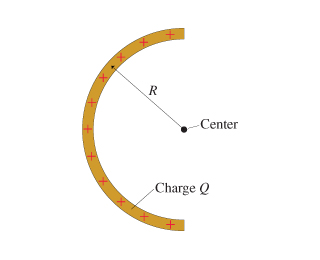# Problem: The figure shows a thin rod with charge Q that has been bent into a semicircle of radius R.Find an expression for the electric potential at the center.Give your answer in terms of Q, R and appropriate constants.

###### FREE Expert Solution

Electric potential, V = kQ/R

88% (483 ratings)###### Problem Details

The figure shows a thin rod with charge Q that has been bent into a semicircle of radius R.Find an expression for the electric potential at the center.

Give your answer in terms of Q, R and appropriate constants.

Frequently Asked Questions

What scientific concept do you need to know in order to solve this problem?

Our tutors have indicated that to solve this problem you will need to apply the Electric Potential concept. You can view video lessons to learn Electric Potential. Or if you need more Electric Potential practice, you can also practice Electric Potential practice problems.

What professor is this problem relevant for?

Based on our data, we think this problem is relevant for Professor Rieger's class at UBC.